### DESIGN AND ANALYSIS OF ALGORITHMS–Question Bank 2012 Edition

Anna University

CS2251 DESIGN AND ANALYSIS OF ALGORITHMS

QUESTION BANK

2012 Edition

UNIT – I

PART A(2MARKS)

1. What is an algorithm?

2. What is meant by open hashing?

3. Define Ω-notation

4.Define order of an algorithm.

5. Define O-notation

6. Define conditional big-oh notation.

7. What do you mean by best case efficiency?

8. What is meant by worst case?

9. Define average case efficiency.

10. Why space complexity of a program is necessary?

11. What is an algorithm design technique?

12. Define little-oh notation.

13. Compare the order of growth n! and 2!

14. What are the types of algorithm efficiencies?

15. Prove or disprove if t(n) E O(g(n))then g(n) E omega t(n)?

PART B(16 MARKS)

1.(i) Explain the various criteria used for analyzing algorithms.

(ii) List the properties of various asymptotic notations.

2. (i) Explain the necessary steps for analyzing the efficiency of recursive algorithms.

(ii) Write short notes on algorithm visualization.

3. Describe briefly the notations of complexity of an algorithm.

4. (i) What is pseudo-code?Explain with an examples.

(ii) Find the complexity (C(n)) of the algorithm for the worst case ,best case and average case.(evaluate average case complexity for n=3,where n is number of inputs)

5. (i) What are the important problem types focused by the researchers?Explain any two with examples.

(ii) What is empirical analysis of an algorithm?Discuss its strength &weakness?

UNIT II

PART A (2 MARKS)

1. Give an non-recursive algorithm to find out the largest element in a list of n numbers.

2. What is meant by divide &conquer?

3. Give the recurrence relation for divide &conquer.

4. What is meant by greedy algorithm?

5. What is meant by knapsack problem?

6. Define fractional knapsack problem.

7. What is the difference between quicksort and mergesort?

8. Give the algorithm of quick sort.

9. What is binary search?

10. What is the average case complexity of linear search algorithm?

11.Define depth first searching technique.

12. Write the procedure for selection sort.

13. Differentiate dynamic programming and divide and conquer..

14. Give two real time problems that could be solved using greedy algorithm.

15. State the time complexity of bubble sort algorithm.

PART B(16 MARKS)

1.(i) Write a psedocode for divide and conquer algorithm for merging two sorted arrays into a single sorted one.Explain with an example. 8

(ii) Set up and solve a recurrence relation for the number of key comparisions made the above psedo code. 8

2. Design a recursive decrease by-one algorithm for sorting the n real numbers in an array with an examples and also determine the number of key comparisions and time efficiency of an algorithm.

 16 3. (i)Define heap.Explain the properties of heap. 8 (ii) Write a simple example to explain heap sort algorithm. 8 4.(i) Write an algorithm to sort a set of N numbers using insertion sort. 8 (ii) Trace the algorithm for the following set of numbers:20,35,18,8,14,41,3,39. 8 5. (i) Explain the difference between depth first and depth first searches. 8 (ii) Mention any three search algorithm which is referred in general. 8

UNIT III

PART A(2 MARKS)

1. Define dynamic programming.

2. Differentiate between greedy method and dynamic programming.

3. Differentiate between divide and conquer and dynamic programming.

4. Define multistage graph.

5.What is meant by all pairs shortest path problem?

6.Write the running time of 0/1 knapsack problem.

7.Define optimal binary search tree.

8. What is meant by all pairs shortest path problem?

9. Give an application of dynamic programming algorithm.

10. Give the running time of the optimal BST algorithm.

11. Write recurrence relation for 0/1 knapsack problem.

12. Write down the floyds algorithm.

13. What is meant by bottom up dynamic programming?

14. What is meant by travelling salesperson problem?

15. What is the running time of dynamic programming TSP?

Part B (16 MARKS)

1. Describe the travelling salesman poblem and discuss how to solve it using dynamic programming?

2. Solve the all pairs shortest path problem for the diagraph with the weight matrix given below.

 a b c d a 0 ∞ 3 ∞ b 2 0 ∞ ∞ c ∞ 7 0 1 d 6 ∞ ∞ 0

3. Find the optimal binary search tree for the key and probabilities given below.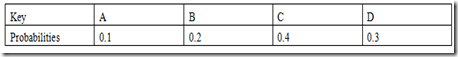4. Find the optimal solution for the given knapsack problem.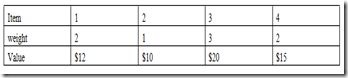5. Solve all pairs shortest path problem for the digraph. 16

b to a -2,a to c-3,c to d-1,c to b-7,d to a- 6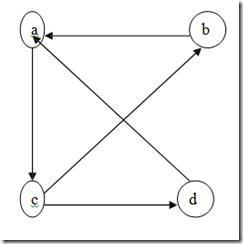UNIT IV

PART A (2marks)

1. State if backtracking always produces optimal solution.

2. Define backtracking.

3. What are the two types of constraints used in backtracking?

4. What is meant by optimization problem?

5. Define Hamiltonian circuit problem.

6. What is Hamiltonian cycle in an undirected graph?

7. Define 8queens problem.

8. List out the application of backtracking.

9. Define promising node and non-promising node.

10. Give the explicit and implicit constraint for 8-queen problem.

11. How can we represent the solution for 8-queen problem?

12. Give the categories of the problem in backtracking.

13. Differentiate backtracking and over exhaustive search.

14. What is state space tree?

15. Find optimal solution for the knapsack instance n =3,w=[20,15,15],P =[40,25,25]and C =30

PART B (16 MARK)

1.Apply backtracking technique to solve the following instance of the subset sum problem S = [1,3,4,5} and d=11 16

2. Explain subset-sum problem and discuss the possible solution strategies using backtracking.

3.Explain N-quence problem with an algorithm.Explain why backtracking is defined as a default procedure of last resort for solving problems.

4. (i) Explain ithe subset-sum problem in detail by justifying it using backtracking algorithm. 8 (ii) Apply backtracking to the problem of finding a Hamiltonian circuit for the following graph.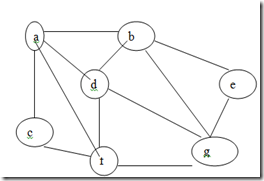5. What is backtracking?Explain in detail.

UNIT- V

PART A(2 MARKS)

1. What is heuristics?

2. Explain briefly branch and bound technique for solving problems.

3. Differentiate between DFS and BFS.

4. What is travelling salesperson problem?

5. What is the formula used to find upper bound for knapsack problem?

6. Differentiate between back tracking and branch and bound.

7. Define articulation point.

8. Define spanning tree.

9. List out the application of branch and bound technique.

10. What is the assignment problem?

11.What is tree edge and cross edge?

12.Define back edge and tree edge.

13.What is the real time application of the assignment problem?

14. What is the metric used to measure the accuracy of approximation of algorithm?

15. What is pre-structuring ?Give examples.

PART B (16 MARK)

1.Solve the following instance of the knapsack problem by the branch and bound algorithm.

 Item Weight Value 1 4 \$40

 2 7 #42 3 5 \$25 4 3 \$12

The Knapsack’s capacity W=10

2. Discuss the solution for knapsack problem using branch and bound technique.

3. What is branch and bound technique?Explain how knapsack problem could be solved using branch and bound technique.Solve the following instance of the knapsack problem by branch and bound algorithm for W=16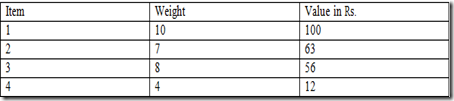4.What is branch and bound?Explain in detail. 16

 Job1 Job2 Job3 Job4 A 9 2 7 8 B 6 4 3 7 C 5 8 1 8 D 7 6 9 4

5. Consider the below matrix for assignment problem involving persons and jobs.Explain in detail how branch and bound technique is useful in solving assignment problems.